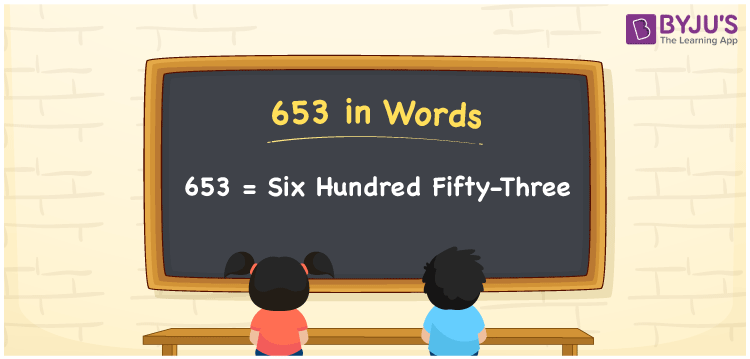# 653 in Words

653 in words is written as Six hundred fifty-three. In both the International System of Numerals and the Indian System of Numerals, 653 is written as Six hundred fifty-three. The number 653 is a Cardinal Number as it represents some quantity. For example, “She spent 653 rupees”.

 653 in Words Six hundred fifty-three Six hundred fifty-three in Number 653

## 653 in English Words

We write 653 in English Words using the letters of the English alphabet. Therefore, we read 653 in English as “Six hundred fifty-three.”## How to Write 653 in Words?

To write 653 in words, we shall use the place value chart. In the place value chart, write 6 in the hundreds, 5 in the tens, and 3 in the ones, respectively. Now let us make a place value chart to write the number 653 in words.

 Hundreds Tens Ones 6 5 3

Thus, we can write the expanded form as

6 × Hundred + 5 × Ten + 3 × One

= 6 × 100 + 5 × 10 + 3 × 1

= 600 + 50 + 3

= 653

= Six hundred fifty-three.

653 is a natural number, the successor of 652 and the predecessor of 654.

653 in words – Six hundred fifty-three

• Is 653 an odd number? – Yes
• Is 653 an even number? – No
• Is 653 a perfect square number? – No
• Is 653 a perfect cube number? – No
• Is 653 a prime number? – Yes
• Is 653 a composite number? – No

## Frequently Asked Questions on 653 in Words

Q1

### How to write 653 in words?

653 in words is written as Six hundred fifty-three.
Q2

### How to write 653 in the International and Indian System of Numerals?

In both, the system of numerals, 653 in words, is written as Six hundred fifty-three.
Q3

### What is the number that comes before 653?

The number that comes before 653 is 652.